# JS循环学习：for循环语句的使用（示例详解）for循环会将控制循环次数的变量预先定义在for语句中，因此for循环语句能够按照已知的循环次数进行循环操作，适用于明确知道脚本需要运行的次数的情况。

for 循环的语法格式如下：

```for (初始化语句; 循环条件; 变量更新--自增或自减) {
语句块;
}```

for循环语句可以拆解为4个部分：`()`号中的三个表达式和`{}`中的“语句块”，下面我们来分析一下。

• 初始化语句（表达式1）：主要是初始化一个变量值，用于设置一个计数器，即循环开始的值；该语句仅在第一次循环时执行，以后都不会再执行。

• 循环条件（表达式2）：循环执行的限制条件，用于控制是否执行循环体中的代码；如果条件为TRUE，则循环继续，如果条件为FALSE ，则循环结束，立即退出循环。

• 变量更新（表达式3）：一个带有自增或自减操作的表达式，循环每执行一次，马上修改计数器的值，以使循环条件逐渐变得“不成立”。

• 语句块：条件判断为真时，需要执行的若干代码。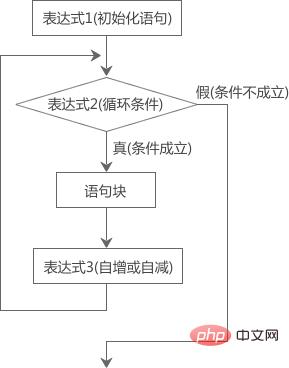```<script type="text/javascript">
var sum=0;
for(var i=1; i<=100; i++){
sum+=i;
}
console.log('1 + 2 + 3 +...+ 99 + 100 = '+sum);
</script>```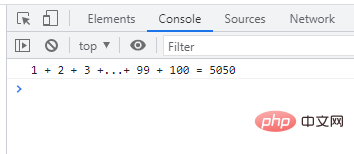for 循环中的三个表达式

JS for 循环中括号中的三个表达式是可以省略的，但是用于分隔三个表达式的分号不能省略，如下例所示：

```// 省略第一个表达式
var i = 0;
for (; i < 5; i++) {
// 要执行的代码
}
// 省略第二个表达式
for (var y = 0; ; y++) {
if(y > 5){
break;
}
// 要执行的代码
}
// 省略第一个和第三个表达式
var j = 0;
for (; j < 5;) {
// 要执行的代码
j++;
}
// 省略所有表达式
var z = 0;
for (;;) {
if(z > 5){
break;
}
// 要执行的代码
z++;
}```

```for (初始化语句1; 循环条件; 变量更新--自增或自减) {
//语句块1;
for (初始化语句2; 循环条件; 变量更新--自增或自减) {
//语句块2;
for (初始化语句3; 循环条件; 变量更新--自增或自减) {
//语句块3;
.....
}
}
}```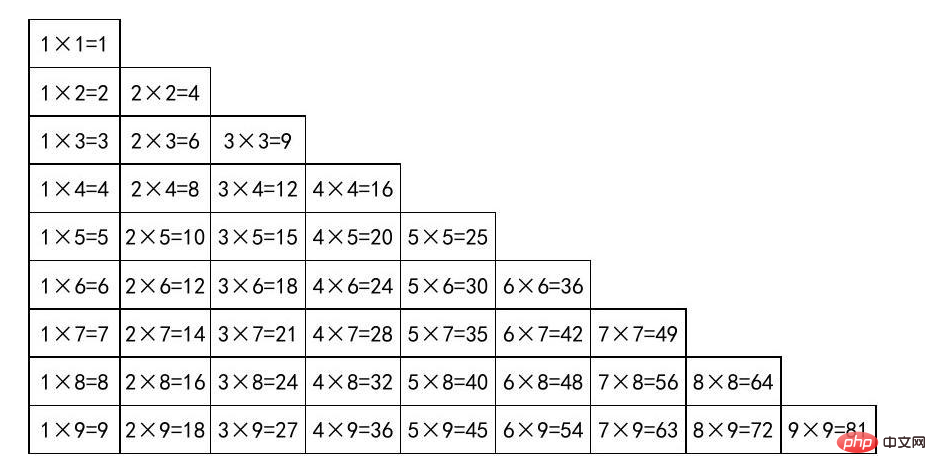• 总共有9行9列，第几行就有几个表达式。

• 第 i 行，表达式就从 i*1 开始，一直到 i*i 结束，共有 i 个表达式（这个效果我们可以通过一次循环实现）。

```for(var i = 1; i <= 9; i++){     //外层循环控制行
for(var j = 1; j <= i; j++) //内层循环控制列
{
document.write(j+"*"+i+"="+j*i+"&nbsp;&nbsp;&nbsp;");
}
document.write("</br>");
}```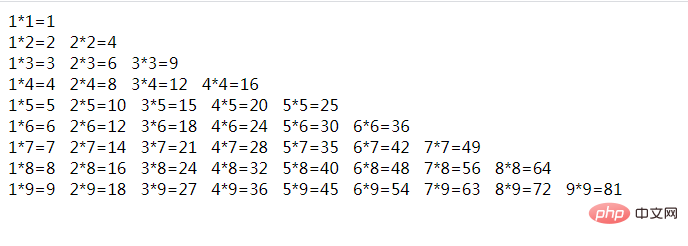```document.write("<table>");

for (var i = 1; i <= 9; i++) { //外层循环控制行
document.write("<tr>");
for (var j = 1; j <= i; j++) //内层循环控制列
{
document.write("<td>" + j + "*" + i + "=" + j * i + "</td>");
}
//换行，控制每行的输出几个表达式
document.write("</tr>");
}
document.write("</table>");```

```table {
width: 600px;
border-collapse: separate;
}

table td {
border: #000 1px solid;
text-align: center;
}```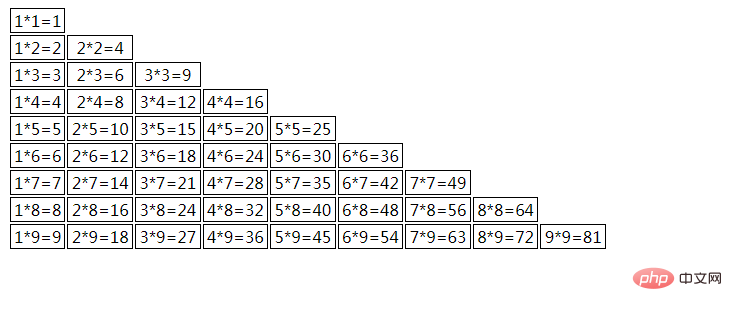【推荐学习：javascript高级教程